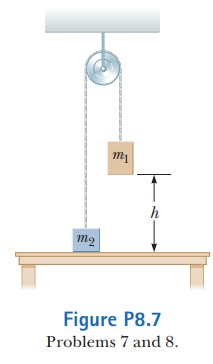# Problem: Two objects are connected by a light string passing over a light, frictionless pulley as shown in Figure P8.7. The object of mass m1 is released from rest at height h above the table. Using the isolated system model, (a) determine the speed of m2 = 4.0 kg just as m1 = 12.0 kg hits the table. Mass, m1 is initially at a height of 2.0 m.(b) find the maximum height above the table to which m2 rises.

###### FREE Expert Solution

Potential energy:

$\overline{){\mathbf{P}}{\mathbf{E}}{\mathbf{=}}{\mathbf{m}}{\mathbf{g}}{\mathbf{h}}}$

88% (227 ratings)###### Problem Details

Two objects are connected by a light string passing over a light, frictionless pulley as shown in Figure P8.7. The object of mass m1 is released from rest at height h above the table.

Using the isolated system model,(a) determine the speed of m= 4.0 kg just as m1 = 12.0 kg hits the table. Mass, m1 is initially at a height of 2.0 m.

(b) find the maximum height above the table to which m2 rises.

Frequently Asked Questions

What scientific concept do you need to know in order to solve this problem?

Our tutors have indicated that to solve this problem you will need to apply the Energy in Connected Objects (Systems) concept. You can view video lessons to learn Energy in Connected Objects (Systems). Or if you need more Energy in Connected Objects (Systems) practice, you can also practice Energy in Connected Objects (Systems) practice problems.

What professor is this problem relevant for?

Based on our data, we think this problem is relevant for Professor Moussa's class at UCF.

What textbook is this problem found in?

Our data indicates that this problem or a close variation was asked in Physics for Scientists and Engineers - Serway Calc 9th Edition. You can also practice Physics for Scientists and Engineers - Serway Calc 9th Edition practice problems.